Next: Gain Saturation Up: PSPC Gain Previous: Spatial Variations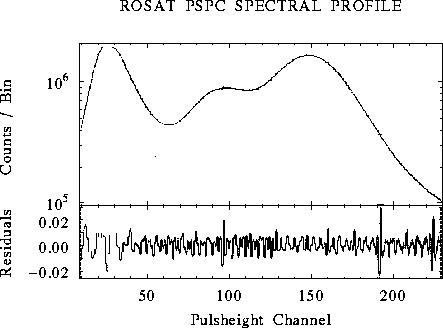Figure 3.11: Upper panel: The cumulative spectrum from all PSPC ground calibration data (processed up to1990 March 17) fitting with a smooth spline function (upper panel) assuming no ADC nonlinearities. The corresponding residuals (lower panel) demonstrate the ADC (pulse height) bins do not have equal widths though on average they are linear to within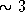%. The residuals are used to determine the true channel boundaries, and thus enable individual events to be corrected for the ADC nonlinearities [Hasinger and Snowden1990].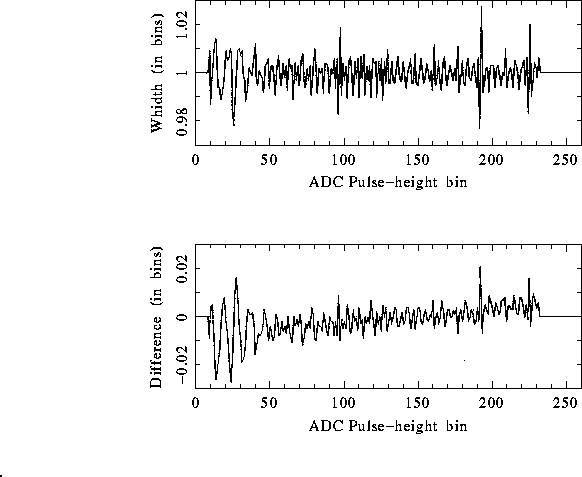Figure 3.12: Upper panel: The width (in channels) of each ADC pulse height bin (cf. the lower panel of Fig. 3.11). Lower panel: The difference between the upper bound of each pulse height channel assuming a linear ADC relationship and that derived from the smooth spline fit to ground calibration data. Both plots are derived from the data file used by SASS5_0 to correct for the nonlinearities in the ADC (cf. the lower panel of Fig. 3.11).

The analog-to-digital converter (ADC) shows nonlinearities in bin width of <3% per channel (although on average they are linear). The effective channel width has been calibrated by performing a spline fit to a cumulative spectrum of all ground calibration data accumulated by1990 March (events, Fig. 3.11) and subtracting a smooth spline function (see Fig. 3.12). The nonlinearity is corrected by drawing a random real number, RAN (between 0 and 1.0), within the non-equidistant channel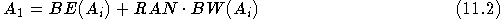where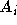&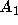are the measured (integer) & corrected (real) pulse height bins, and BE & BW arrays containing the (lower) edge and width of the 256 non-equidistant channels respectively (see also Chap. 8).

If you have problems/suggestions please send mail to rosat_svc@mpe-garching.mpg.de